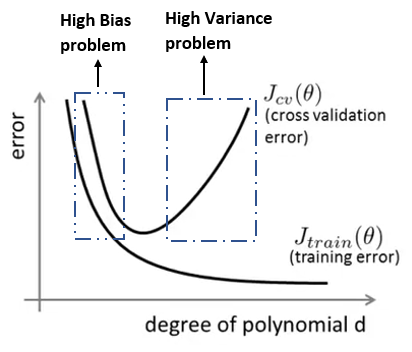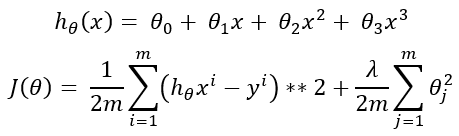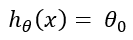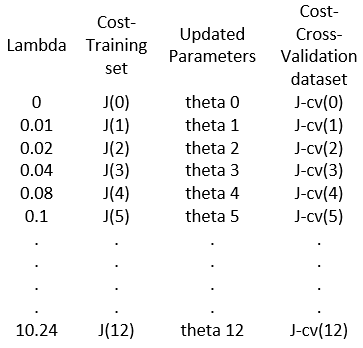# How to Improve a Machine Learning Algorithm: RegularizationThis article explains the reason for the poor performance of a machine learning algorithm and how to improve it. If the machine learning algorithm does not work as well as you expected, almost all the time it happens because of bias or variance. The algorithm may be suffering from either underfitting or overfitting or a bit of both.

If the machine learning algorithm does not work as well as you expected, almost all the time it happens because of bias or variance. The algorithm may be suffering from either underfitting or overfitting or a bit of both. It’s important to figure out the problem to improve the algorithm.

## Bias vs Variance

Think of polynomial regression. As we know if we increase the degree of the polynomial, accuracy goes higher. But this accuracy is on the training set. If the degree of the polynomial is high enough, the algorithm learns the training data so well that it can fit in the training dataset perfectly. Look at the picture below. Higher the degree of the polynomial, the lower the training error becomes.Source: Author

Cross-validation data has an interesting part to play here. When the degree of the polynomial is lower, Both training errors and the validation errors will be high. This is called a high bias problem. You can call it an underfitting problem as well. So, the sign of a high bias problem is, the training set accuracy and the validation set accuracy both are low.

On the other hand, when the degree of the polynomial is too high, training data will fit too well in the algorithm. So, the training error will be very low. But the algorithm will perform very poorly on the cross-validation data. So, the cross-validation error will be very high. This is called a high variance problem or an overfitting problem. The sign of an overfitting problem or a high variance problem is, the training set accuracy will be very high and the cross-validation set accuracy will be poor.

## Regularization

Regularization helps to deal with overfitting or underfitting problem. Choosing the regularization parameter lambda can be critical.Here is the equation for the hypothesis (on top) and the cost function (at the bottom) for polynomial regression. If we choose too large of a lambda such as 10000, the theta values except for theta0 will be insignificant. Because all the theta values are randomly initialized values that are the values between 0 to 1. In that case, the hypothesis will be:As a result, we will have a high bias (underfitting) problem. If the lambda is too small, in a higher-order polynomial, we will get a usual overfitting problem. So, we need to choose an optimum lambda.

## How to Choose a Regularization Parameter

It is worth spending some time to choose a good regularization parameter. We need to start by taking a few lambda values starting from zero. Here is the step by step process:

1. Choose some lambda values such as 0, 0.02, 0.04, 0.08, 0.1, …. 10.24.
2. Use these lambdas and train the model using the training set and minimize the cost. So, we will get a minimized cost and theta values for each lambda value.
3. Use the optimized theta values and calculate the cost functions for the cross-validation dataset.
4. Find out which lambda value gave the smallest cost in the cross-validation set. That lambda value should be our final regularization parameter. In the chart below, say, j-cv(3) is the smallest. The final regularization parameter lambda will be 0.04. In this chart, I tried to list all the steps.## Artificial Intelligence vs. Machine Learning vs. Deep Learning

Simple explanations of Artificial Intelligence, Machine Learning, and Deep Learning and how they’re all different

## Comparison of Data Science Vs Machine Learning Vs Artificial Intelligence

Explore the differences between Data Science, Machine Learning, Artificial Intelligence. Understand how DS, ML, and AI is extremely inter-related. Choose the Right career path!

## 10 Data Science, Machine Learning and AI Podcasts You Must Listen To

10 Data Science, Machine Learning and AI Podcasts You Must Listen To - With rapid changes in data science, machine learning and artificial intelligence, podcasts are a great way to keep yourself updated with new developments.

## Learn Data Science Today - Data Science Tutorial for Beginners 2020!

How and why to start Learning to be a data scientist in 2020! This Data Science Course will give you a Step by Step idea about the Data Science Career, Data science Hands-On Projects, roles & salary offered to a Data Scientist!

## AI on Pause? Artificial intelligence (AI)

Pretty much all AI/ML customer and transactional models have been on pause for the last few months. As we speak, history is being written…